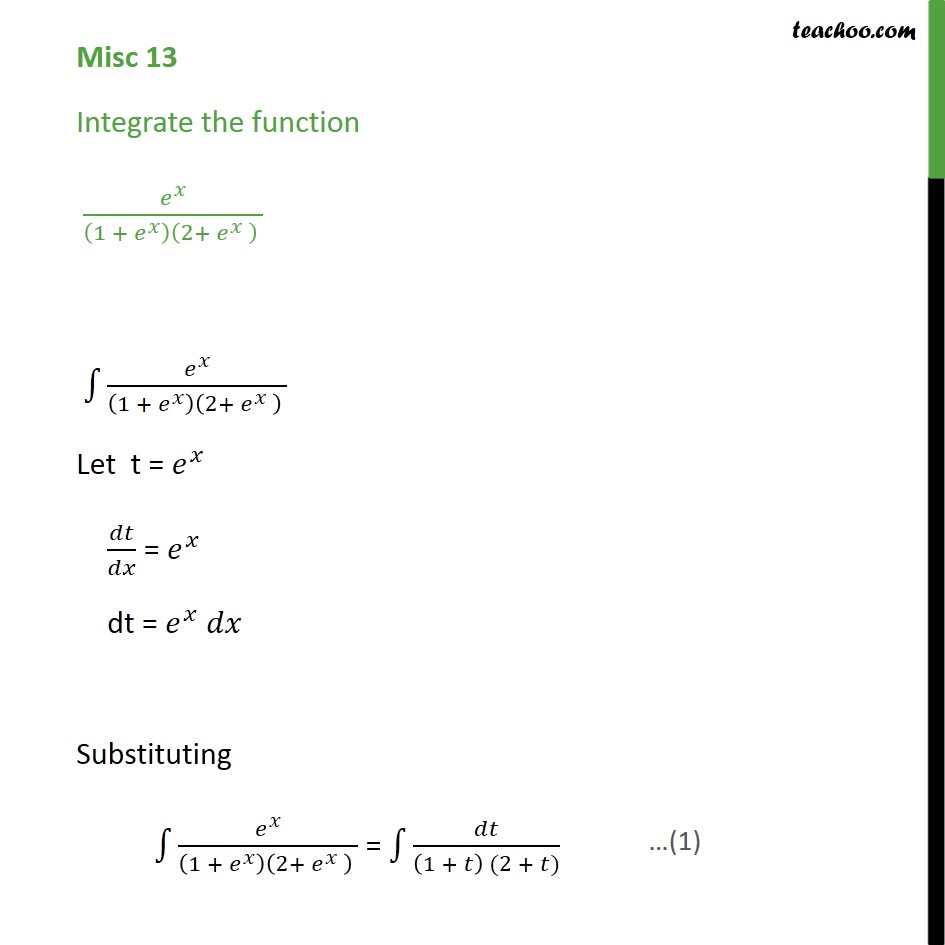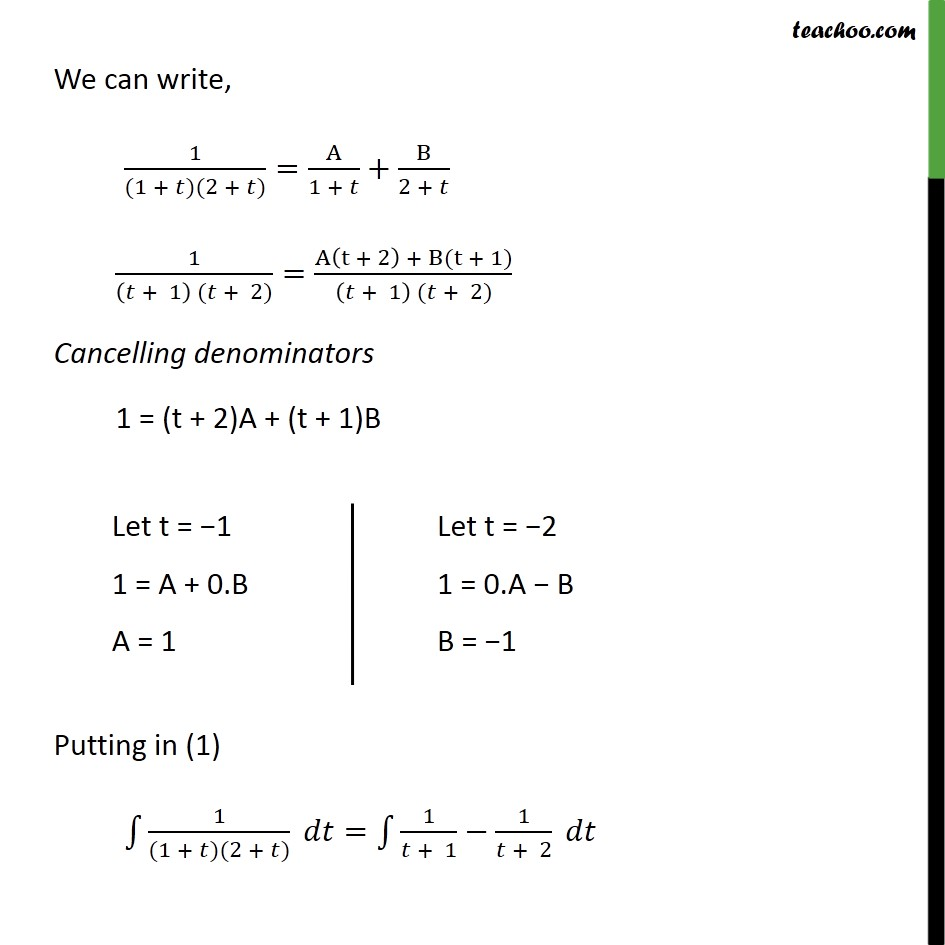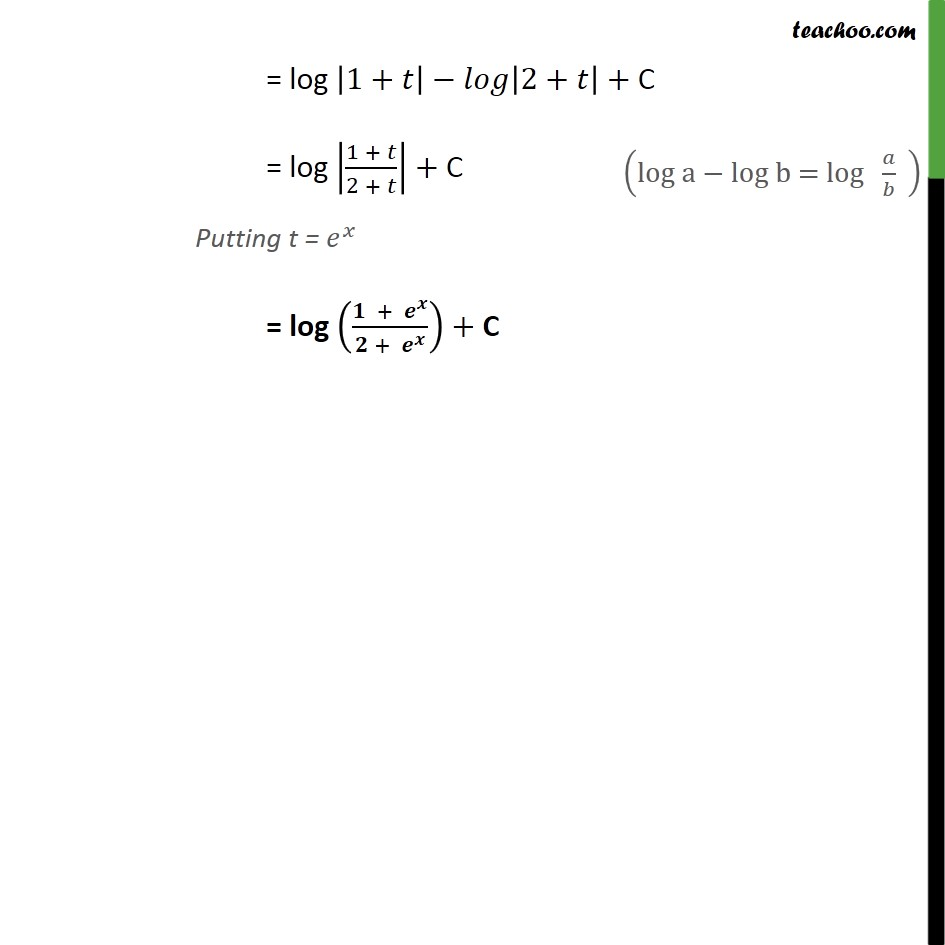Integration by partial fraction - Type 1

Chapter 7 Class 12 Integrals
Concept wiseIntroducing your new favourite teacher - Teachoo Black, at only ₹83 per month

### Transcript

Misc 13 Integrate the function ^ /((1 + ^ )(2+ ^ ) ) 1 ^ /((1 + ^ )(2+ ^ ) ) Let t = ^ / = ^ dt = ^ Substituting 1 ^ /((1 + ^ )(2+ ^ ) ) = 1 /((1 + ) (2 + )) We can write, 1/((1 + )(2 + ))=A/(1 + )+B/(2 + ) 1/(( + 1) ( + 2))=(A(t + 2) + B(t + 1))/(( + 1) ( + 2)) Cancelling denominators 1 = (t + 2)A + (t + 1)B Putting in (1) 1 1/((1 + )(2 + )) = 1 1/( + 1) 1/( + 2) = log |1+ | |2+ |+ C = log |(1 + )/(2 + )|+ C Putting t = ^ = log (( + ^ )/( + ^ ))+ C